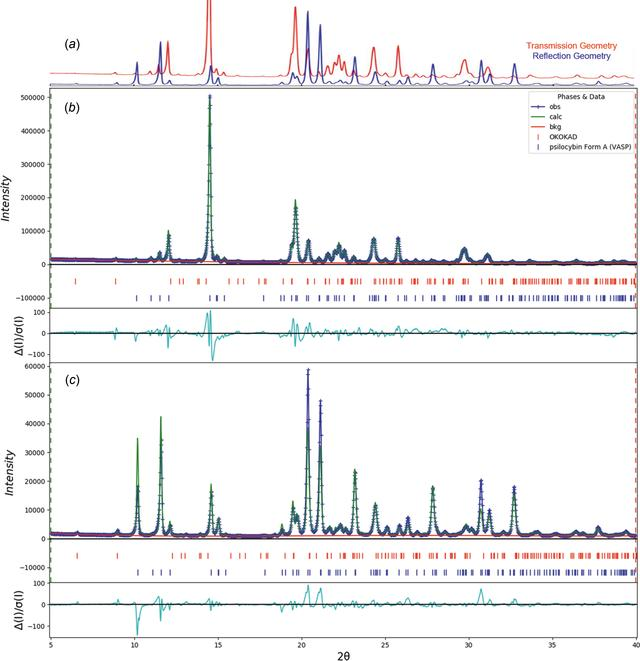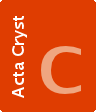disable zoom     view article Figure 20 (a) Comparison of PXRD patterns for Sample 4 collected by transmission geometry (red) and reflection-based configurations (blue). (b) The Rietveld plot for the refinement of psilocybin Sample 4 (Hydrate A and Polymorph A) using transmission data. The blue crosses represent the observed data points and the green line is the calculated pattern. The cyan curve is the normalized error plot obtained by transmission geometry. (c) The Rietveld plot for the refinement of psilocybin Sample 4 (Hydrate A and Polymorph A) using reflection data. The blue crosses represent the observed data points and the green line is the calculated pattern. The cyan curve is the normalized error plot.STRUCTURALCHEMISTRY
ISSN: 2053-2296
Volume 78| Part 1| January 2022| Pages 36-55